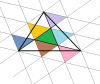BrainDen.com - Brain Teasers
• 0

# A triangle defined by cevians

## Question

Given triangle ABC, with cevians AD, BE, CF dividing the sides in ratio 1:2, a smaller triangle forms in the middle.  What is the area of the triangle in the middle?

## Recommended Posts

• 0

I gave up on finding a rigorous proof.

My 1/7 answer was based on a carefully drawn figure and a guess that the ratio might be integral.

I thought I could do the analysis for an equilateral triangle but I gave up even on that case.

Any triangle can be affinely transformed (and thus preserve relative areas) into equilateral.

Instead, I found a formula (without derivation) in some obscure online class notes.

If cevians cut opposite sides into fractions p and q you get the fractional area of the inside triangle as:

Area (inside triangle) / Area (large triangle) = { p1p2p3 - q1q2q3 }2 / { (p1 + q2q3) (p2 + q3q1) (p3 + q1q2) }

It's not difficult to plug in 1/3 and 2/3 respectively for all the p and q to get 1/7 as a ratio.

Interestingly, there is one other set of p and q values that leads an integer ratio.

Side ratios of 2/5 and 3/5 lead, surprisingly, to an area ratio of 1/19.

##### Share on other sites

• 0

Suppose ABC has area of 1.

Cevians divide areas in proportion to lengths on the opposite side.

That is given as 1/3, so the cevians define three surrounding triangles that have area of 1/3.

However, they overlap each other near the vertices.

The area of the inner triangle is thus given by the sum of these overlaps.

The cevians themselves are divided into segments that appear to have ratios of 1:3:3.

If so, then 1/7 of each surrounding triangle is overlapped.

The central triangle thus has area of 3 x (1/3) x (1/7) = 1/7.

There must be an obvious way to show the 1:3:3 cevian proportions, but I don't see it.

##### Share on other sites

• 0

I assumed each vertex was the intersection of a 1/3 side and a 2/3 side.
The figure makes the most sense that way.

Edit::

I see now that the cevians are divided into 3/7 3/7 and 1/7 segments.

It's too verbose a process without a figure, which I may construct later,

but I'm convinced of the 1/7 for the area of the inner triangle.

Edited by bonanova
##### Share on other sites

• 0I would consider the total area of the triangle using the smallest triangle as a referent for the others.

take advantage of symetry

##### Share on other sites

• 0

Hmmm 1/19 is what I found.

I gave up on finding a rigorous proof.

My 1/7 answer was based on a carefully drawn figure and a guess that the ratio might be integral.

I thought I could do the analysis for an equilateral triangle but I gave up even on that case.

Any triangle can be affinely transformed (and thus preserve relative areas) into equilateral.

Instead, I found a formula (without derivation) in some obscure online class notes.

If cevians cut opposite sides into fractions p and q you get the fractional area of the inside triangle as:

Area (inside triangle) / Area (large triangle) = { p1p2p3 - q1q2q3 }2 / { (p1 + q2q3) (p2 + q3q1) (p3 + q1q2) }

It's not difficult to plug in 1/3 and 2/3 respectively for all the p and q to get 1/7 as a ratio.

Interestingly, there is one other set of p and q values that leads an integer ratio.

Side ratios of 2/5 and 3/5 lead, surprisingly, to an area ratio of 1/19.

##### Share on other sites

• 0

Hmmm 1/19 is what I found.

A rough sketch for 1:2 split rules that out.

Since 1/19 is the result for a 2:3 split, maybe there's a slip in how the ratio was used in the calculations.

Try using a 1:1 ratio, and see if that calculates 0 area.

You've done more analysis than I have.##### Share on other sites

• 0

another proof1) draw 3 additional lines parallel to each cevian passing through the remaining vertices of 2 triangles.

2) show that 6 other shaded triangles are mirror images of the green triangle and therefore have the same area

3) show that every part of a shaded triangle that lies outside of the big triangle has an equal unshaded part inside the big triangle. Midpoints of big triangle's sides are points of symmetry.

Edited by k-man
•1
##### Share on other sites

• 0

The 2/5-3/5 <==> 1/19 case probably admits to this proof as well.

There would be 18 similar triangles instead of 6.

## Join the conversation

You can post now and register later. If you have an account, sign in now to post with your account.×   Pasted as rich text.   Paste as plain text instead

Only 75 emoji are allowed.

×   Your previous content has been restored.   Clear editor

×   You cannot paste images directly. Upload or insert images from URL.